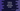# How to convert a python dictionary to list## Python program to convert a dictionary to list:

Python dictionary is used to hold key-value pairs. We can’t directly conver a list to a dictionary. But, we can use other dictionary methods those can be used to get the dictionary items in a list.

This post will show you how to convert a dictionary to list in python.

### Using keys():

keys() is a dictionary method and it returns a view object that holds the keys of the dictionary as a list.

Below is the syntax of keys():

``dict.keys()``

It doesn’t take any parameters. We can use list() method to convert them to a list.

Below is a complete example of keys():

``````days_dict = {
1: "Sun",
2: "Mon",
3: "Tues",
4: "Wed",
5: "Thurs",
6: "Fri",
7: "Sat"
}

keys_days_dict = days_dict.keys()
list_keys = list(keys_days_dict)

print(keys_days_dict)
print(list_keys)``````

It will print the below output:

``````dict_keys([1, 2, 3, 4, 5, 6, 7])
[1, 2, 3, 4, 5, 6, 7]``````

As you can see here, the second print statement prints a list holding the keys.

### Using values():

values() is similar to keys(). It returns a view object that holds the values of a dictionary as a list. We can use list() to convert them to a list.

Below is the syntax of values():

``dict.values()``

Below example uses the same dictionary as the above example:

``````days_dict = {
1: "Sun",
2: "Mon",
3: "Tues",
4: "Wed",
5: "Thurs",
6: "Fri",
7: "Sat"
}

values_days_dict = days_dict.values()
list_keys = list(values_days_dict)

print(values_days_dict)
print(list_keys)``````

It will print:

``````dict_values(['Sun', 'Mon', 'Tues', 'Wed', 'Thurs', 'Fri', 'Sat'])
['Sun', 'Mon', 'Tues', 'Wed', 'Thurs', 'Fri', 'Sat']``````

### Using items():

items() is another method we can use. It returns a view object that contains a list of key-value tuple pairs. So, using list(), we can create a list of key-value pairs.

``````days_dict = {
1: "Sun",
2: "Mon",
3: "Tues",
4: "Wed",
5: "Thurs",
6: "Fri",
7: "Sat"
}

items_days_dict = days_dict.items()
list_keys = list(items_days_dict)

print(items_days_dict)
print(list_keys)``````

It will print:

``````dict_items([(1, 'Sun'), (2, 'Mon'), (3, 'Tues'), (4, 'Wed'), (5, 'Thurs'), (6, 'Fri'), (7, 'Sat')])
[(1, 'Sun'), (2, 'Mon'), (3, 'Tues'), (4, 'Wed'), (5, 'Thurs'), (6, 'Fri'), (7, 'Sat')]``````